# Advanced Algebra Trigonometry Objective SWBAT solve linear equations

• Slides: 11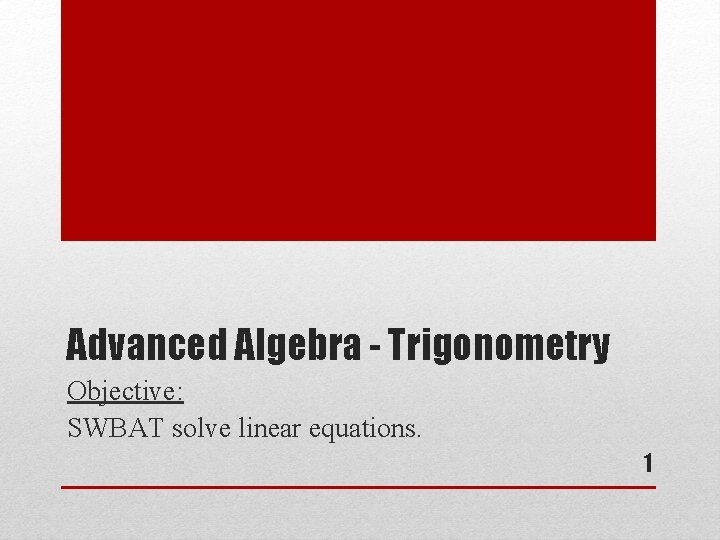Advanced Algebra - Trigonometry Objective: SWBAT solve linear equations. 1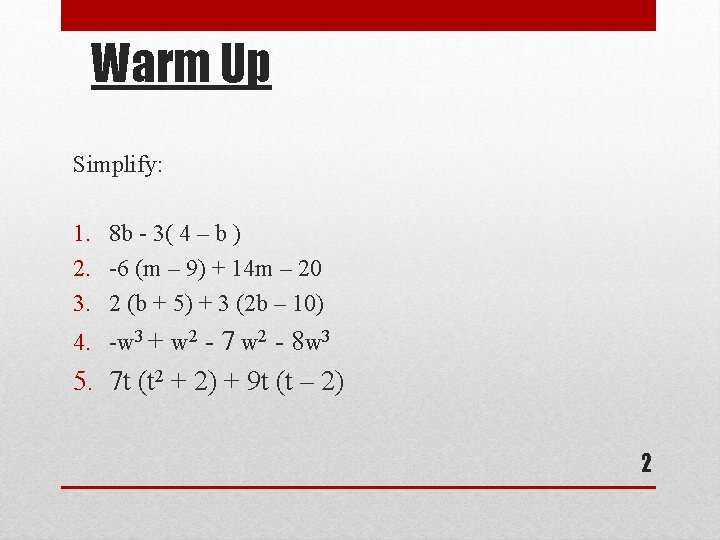Warm Up Simplify: 1. 8 b - 3( 4 – b ) 2. -6 (m – 9) + 14 m – 20 3. 2 (b + 5) + 3 (2 b – 10) 4. -w 3 + w 2 - 7 w 2 - 8 w 3 5. 7 t (t 2 + 2) + 9 t (t – 2) 2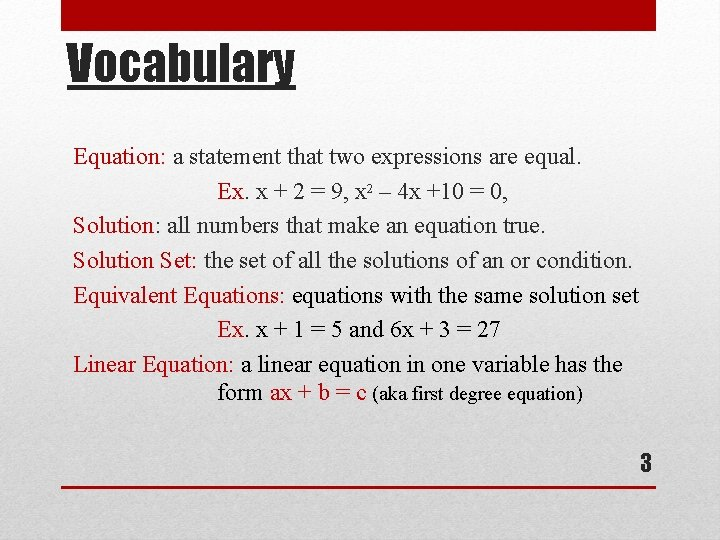Vocabulary Equation: a statement that two expressions are equal. Ex. x + 2 = 9, x 2 – 4 x +10 = 0, Solution: all numbers that make an equation true. Solution Set: the set of all the solutions of an or condition. Equivalent Equations: equations with the same solution set Ex. x + 1 = 5 and 6 x + 3 = 27 Linear Equation: a linear equation in one variable has the form ax + b = c (aka first degree equation) 3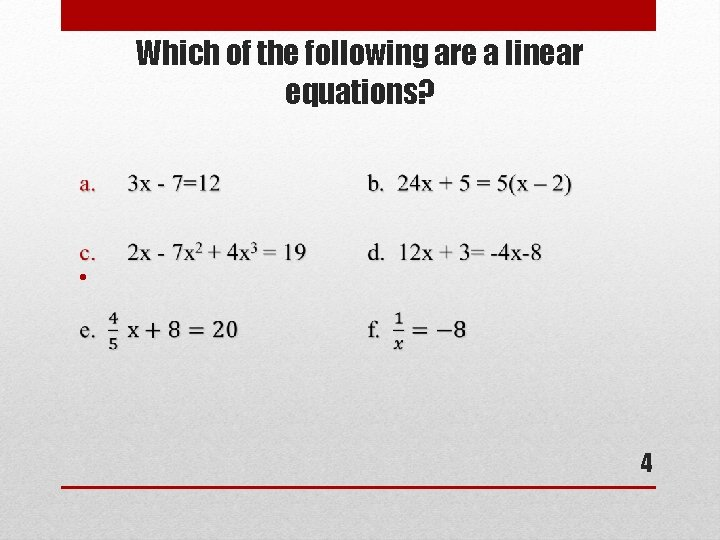Which of the following are a linear equations? • 4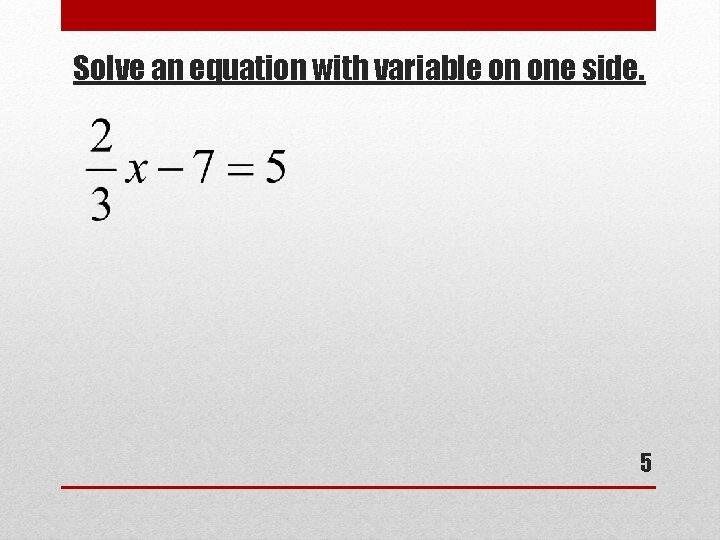Solve an equation with variable on one side. 5Solve an equation with variable on both sides. Solve: 8 y – 16 = 13 y + 9 6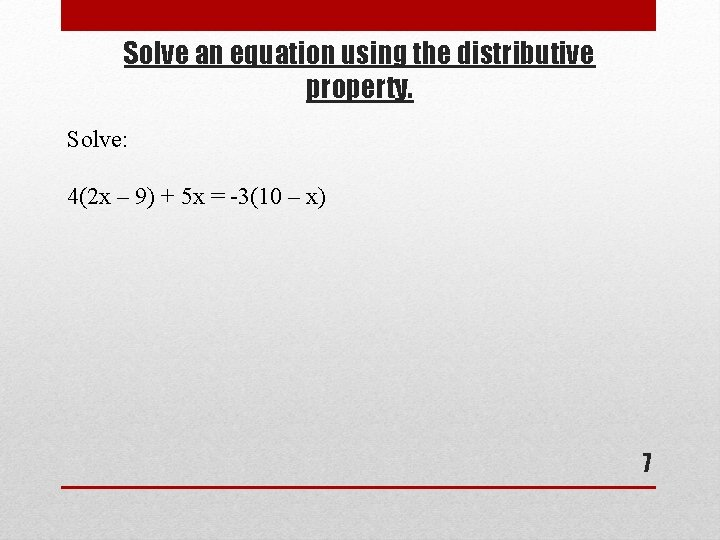Solve an equation using the distributive property. Solve: 4(2 x – 9) + 5 x = -3(10 – x) 7Solve an equation with clearing a fraction. Solve: 8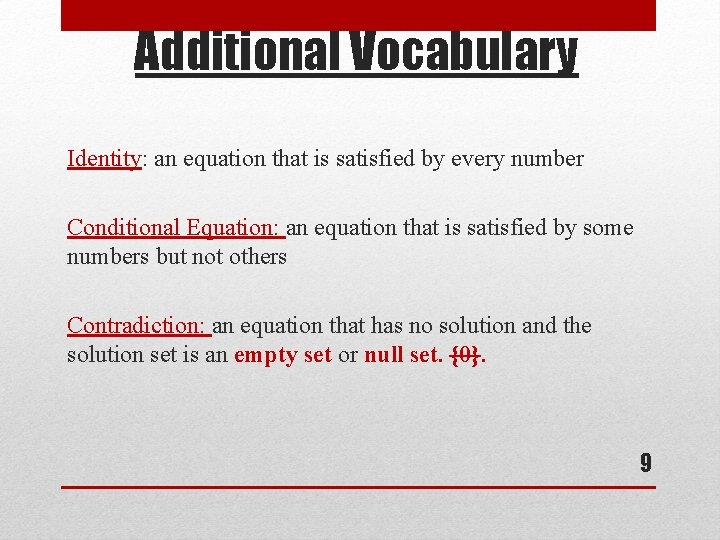Additional Vocabulary Identity: an equation that is satisfied by every number Conditional Equation: an equation that is satisfied by some numbers but not others Contradiction: an equation that has no solution and the solution set is an empty set or null set. {0}. 9Identify the Type of Equation. Decide whether each equation is an identity, a conditional equation, or a contradiction. Give the solution set. a. -2(x + 4) + 3 x = x – 8 b. 5 x – 4 = 11 c. 3(3 x-1) = 9 x +7 10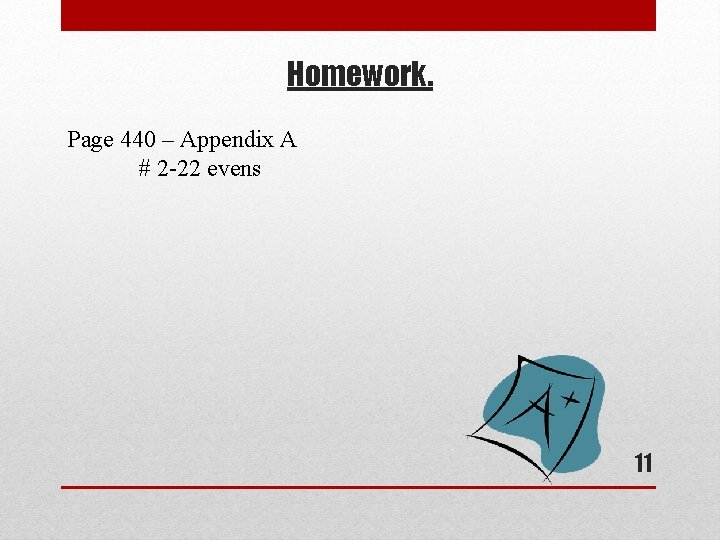Homework. Page 440 – Appendix A # 2 -22 evens 11